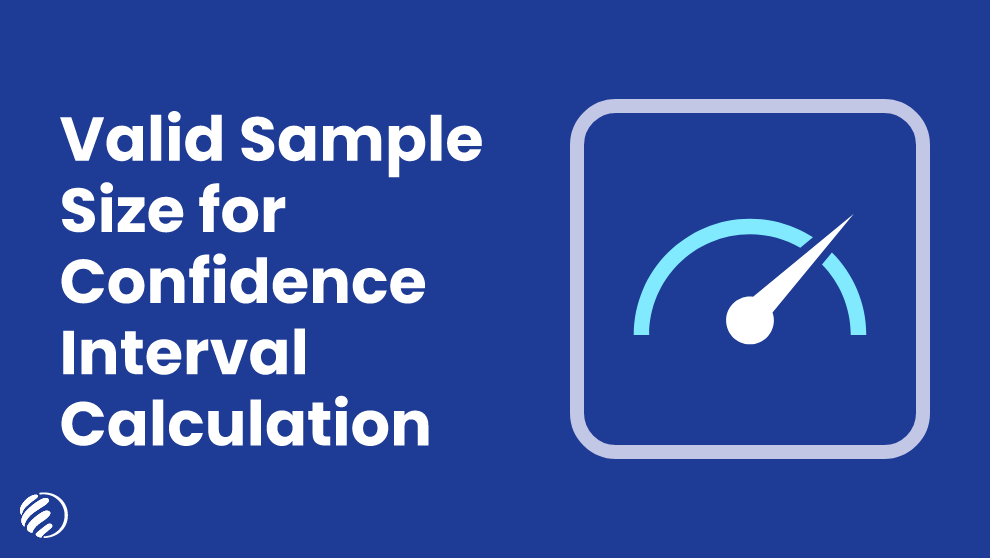# Valid Sample Size for Confidence Interval Calculation4300 Views8 Mins To ReadCategory: CalculatorsSeptember 11, 2021

The confidence interval calculation involves several intricacies for statisticians. One of the major problems faced by people is the determination of a valid sample size for calculating accurate results and completing a survey. The issue mainly arises for people who haven’t studied stats in college or university, or the calculation method has entirely vanished from their memory. If you also have the same query, then this blog is mainly for you. We are here to discuss the easy way of choosing a valid sample size for confidence interval calculation. You don’t need to panic or go through your study books again to learn about sample size, as we have covered everything in this blog. So without any further ado, let’s get started!

## What is the Accurate Sample Size for Decision Making?

While analyzing a particular experiment or research, the accuracy of sample size plays a major role in the decision-making process. The enormity of data allows the researchers to make accurate decisions with less margin of error. However, it doesn’t mean that you always need a large sample size for precise results.

During the comparison of two populations’ means, two different types of tests are used as per the size of the population. If the population size is greater than 30, then the statisticians have to use the Z-test; on the other hand, if the sample size is less than 30, then the t-test is used.

## Role of Confidence Level & Margin of Error

Besides considering the valid sample size for the calculation of confidence intervals of any survey, you also need to understand the role of confidence level and margin of error. These two elements also play a crucial role in finding the intervals between which the true parameter of a population exists. Let’s understand how these two elements affect the confidence interval calculation of any survey.

The confidence level is basically a range that determines the chances of uncertainty involved with an estimation. If you want a higher confidence level, then you need a bigger sample size for conducting this survey. In contrast, the margin of error is the level of accuracy required by the researcher in his/her estimate. The estimation of margin of error is usually taken in percentage, as it reflects the chances of the survey going beyond the confidence intervals.

## Get Precise Results with Confidence Interval Calculator

No matter how complex your data is, you can easily calculate the confidence intervals by entering the amounts in the given fields. The confidence interval calculator available on searchenginereports.net is the best utility you can find over the web to calculate precise results. Our web portal presents a free-of-cost utility to its users and doesn’t impose any restriction on its usage. You can use the confidence interval calculator for conducting as many calculations as you desire. This online utility doesn’t ask you to get registered or access it through a specific device due to its compatibility with all operating systems.

### That’s it!

The validity of the sample size is essential for the calculation of confidence intervals. Instead of following manual methods, you can use the automated tool for calculating confidence intervals in a matter of seconds. You don’t need to rely on professionals’ assistance for conducting this calculation. The user-friendly interface of a confidence interval calculator allows you to get your hands on precise results without any hassle.